# Overdetermined and underdetermined systems of equations put simply

This article aims at providing real world examples and links for overdetermined and undetermined systems of equations. Before starting, we will suppose that all over and underdetermined systems are obtained from square systems which admit one and only one solution (i.e. comes from a coefficient matrix with non zero determinant).

## Overdetermined systems

When a system of linear equations has more equations than unknowns, we say it is overdetermined. It means what it says: too many rules at once are being imposed, and some of them may be conflicting. Still, it is false to say that an overdetermined system does not have any solutions: it may or it may not.

### Intuition

Intuitively, we can think of a system of equations as a set of requests. Imagine you have a group ofpeople in front of you (the unknowns), and you are supposed to give each person something specific to do. If you give more commands than the number of people, then we have an overdetermined system. It is clear that when this happens, at least one person must have received more than one command. However, there are two possible scenarios.

If you give more orders than people, but the surplus commands are just reformulations of other orders, then this is not a problem, the system does have a solution.

Take this example:

• George, get me one bottle of water
• Lisa, solve that equation
• George, get me two-minus-one bottles of water

There are two people, and we have given three commands. But look, we told George to fetch a water bottle twice! We told him a bit differently the second time, but the meaning was just the same. Thus, we can say the last command is irrelevant. So the idea is that an equation that is proportional to another one already in the system is basically like a game in which the second rule is to respect the first rule!

So if the system had a solution when there were as many commands as people, it still does now, because we can throw the superfluous commands out of the window, since they are unneeded.

Instead, if you give more orders than people, and some commands are conflicting, then the system does not have a solution.

Take this example:

• George, get me one bottle of water
• Lisa, solve that equation
• George, go buy me a swimming pool

George is likely to be confused, and will ask what we want him to do, either to get a water bottle or a swimming pool. The point is that we want George to do both things at the same time. The fact that it is impossible for George to do two things at once expresses the fact that the corresponding system of equations does not have a solution.

In this case, it is just like having a game where the second rule says not to follow the first rule: it is impossible to play a game like that.

Math Example: the simplest overdetermined system is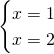which doesn’t have a solution because we are askingto beand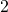at the same time. This is just like asking your neighbor to be male and female at the same time!

## Underdetermined systems

If you give less orders than number of people, then we have an underdetermined system. When this happens, at least one person must have not received any command. This time, the idea is that people who don’t receive any commands are free to do whatever they want.

For example, imagine to have George, Lisa, Bob and Alice in front of you. If your commands are:

• George, get me one bottle of water
• Lisa, solve that equation
• Alice, build a car with those Lego

then Bob hasn’t received any command, and will assume he is free to do whatever he wants.

## Final remarks

However, notice that everything can happen: what appears to be an overdetermined system could actually turn out to be an underdetermined one (see ex. 1) and an overdetermined system could have no solution (see ex. 2).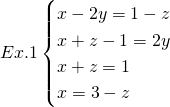in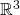An apparently overdetermined system which is actually underdetermined and doesn’t even have a solution.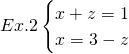inAn overdetermined which doesn’t have a solution.

Finally, notice that in reality commands are usually addressed to more than one person at a time. A system of equations in real life is something like:Here intuition gets trickier, because each command mixes at least two people, and can’t be rendered in natural language. (You can think of knowing what two people should do, but can’t know exactly who should do what without additional information, which is indeed carried by the mathematical expressions.)

Still, the commands analogy is useful in understanding what underdetermined and overdetermined systems are and why they have infinite or no solutions.

1.SHISHIR PANDEY says: